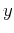Nonhyperbolic reflection moveout of-waves: An overview and comparison of reasonsNext: Bibliography Up: Fomel & Grechka: Nonhyperbolic Previous: Conclusions

# Acknowledgments

This paper is the result of a year-long email correspondence. Its outline was created when the first author visited Colorado School of Mines. We acknowledge the support of the Stanford Exploration Project and the Center for Wave Phenomena (CWP) Consortium Project. The second author was also supported by the United States Department of Energy (Award #DE-FG03-98ER14908). We thank Ken Larner and Petr Jílek for reviewing the manuscript, and Ilya Tsvankin and other members of the A(nisotropy)-team for insightful discussions.

# NORMAL MOVEOUT BEYOND THE NIP THEOREM

In this Appendix, we derive equations that relate traveltime derivatives of the reflected wave, evaluated at the zero offset point, and traveltime derivatives of the direct wave, evaluated in the vicinity of the zero-offset ray. Such a relationship for second-order derivatives is known as the NIP (normal incidence point) theorem (Hubral, 1983; Chernjak and Gritsenko, 1979; Hubral and Krey, 1980). Its extension to high-order derivatives is described by Fomel (1994).

Reflection traveltime in any type of model can be considered as a function of the source and receiver locationsandand the location of the reflection point, as follows:(83)

whereis the midpoint,is the half-offset, and the functionhas a natural decomposition into two parts corresponding to the incident and reflected rays:(84)

whereis the traveltime of the direct wave. Clearly, at the zero-offset point,(85)

wherecorresponds to the reflection point of the zero-offset ray.

Differentiating equation (A-1) with respect to the half-offsetand applying the chain rule, we obtain(86)

According to Fermat's principle, one of the fundamental principles of ray theory, the ray trajectory of the reflected wave corresponds to an extremum value of the traveltime. Parameterizing the trajectory in terms of the reflection point locationand assuming thatis a smooth function of, we can write Fermat's principle in the form(87)

Equation (A-5) must be satisfied for any values ofand. Substituting this equation into equation (A-4) leads to the equation(88)

Differentiating (A-6) again with respect to, we arrive at the equation(89)

Interchanging the source and receiver locations doesn't change the reflection point position (the principle of reciprocity). Therefore,is an even function of the offset, and we can simplify equation (A-7) at zero offset, as follows:(90)

Substituting the expression for the function(A-2) into (A-8) leads to the equation(91)

which is the mathematical formulation of the NIP theorem. It proves that the second-order derivative of the reflection traveltime with respect to the offset is equal, at zero offset, to the second derivative of the direct wave traveltime for the wave propagating from the incidence point of the zero-offset ray. One immediate conclusion from the NIP theorem is that the short-spread normal moveout velocity, connected with the derivative in the left-hand-side of equation (A-9) can depend on the reflector dip but doesn't depend on the curvature of the reflector. Our derivation up to this point has followed the derivation suggested by Chernjak and Gritsenko (1979).

Differentiating equation (A-7) twice with respect toevaluates, with the help of the chain rule, the fourth-order derivative, as follows:(92)

Again, we can apply the principle of reciprocity to eliminate the odd-order derivatives ofin equation (A-11) at the zero offset. The resultant expression has the form(93)

In order to determine the unknown second derivative of the reflection point location, we differentiate Fermat's equation (A-5) twice, obtaining(94)

Simplifying this equation at zero offset, we can solve it for the second derivative of. The solution has the form(95)

Here we neglect the case of, which corresponds to a focusing of the reflected rays at the surface. Finally, substituting expression (A-14) into (A-12) and recalling the definition of thefunction from (A-2), we obtain the equation(96)

which is the same as equation (67) in the main text. Higher-order derivatives can be expressed in an analogous way with a set of recursive algebraic functions (Fomel, 1994).

In the derivation of equations (A-9) and (A-15), we have used Fermat's principle, the principle of reciprocity, and the rules of calculus. Both these equations remain valid in anisotropic media as well as in heterogeneous media, providing that the traveltime function is smooth and that focusing of the reflected rays doesn't occur at the surface of observation.Nonhyperbolic reflection moveout of-waves: An overview and comparison of reasonsNext: Bibliography Up: Fomel & Grechka: Nonhyperbolic Previous: Conclusions

2014-01-27